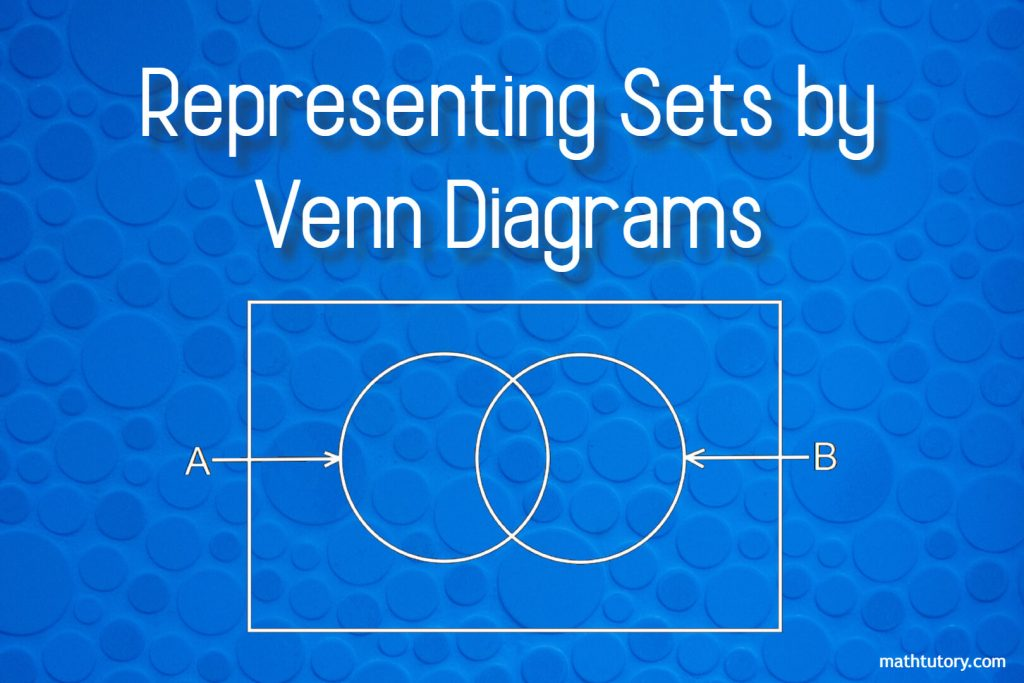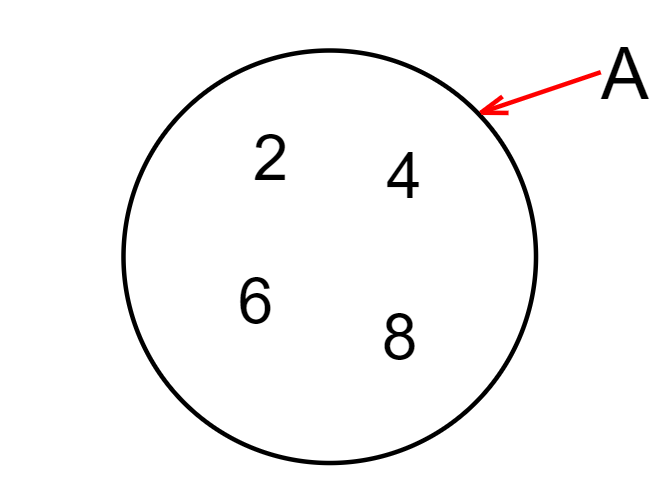# Representing Sets by Venn Diagrams

Venn diagrams are the most common way of representing a set.

This method was introduced by the English mathematician John Venn. Therefore these are called Venn Diagram.

Set is a collection of items that can be clearly identified.
The items in a set are called its elements.

Example:

Let A be the set of even numbers between 0 and 10.
We can represent this as,

01. A = { set of even numbers between 0 and 10}

02. A = { 2, 4, 6, 8 }

We can see that 4 is an element of set A.
So by set notation, we can express it as,

4 ∈ A

and also 7 is not an element of A, we can express it as,

7 ∉ A

## What is a Venn Diagram?

Let’s take the same example

When a set is represented in the above manner by a closed plane figure, (we usually use round or oval shape) such a figure is defined as a Venn diagram.

## Key Features of a Venn Diagram

01. Should be a closed plane figure.

02. The element should not be separated by commas.

03. Elements should be within the closed figure.

04. Name the set by using an arrow.

## Finite and Infinite Sets

Sets with a specific number of elements are known as Finite Sets.

Examples:

A = {Multiples of 3 between 0 and 20}

So when we consider elements we can write this set again as,

A = { 3, 6, 9, 12, 15, 18 }

There are only six elements in this set and through set notation

n ( A ) = 6

n = ( A ) means the number of elements in set A.

Sets that have an infinite number of elements are known as Infinite Sets.

Examples:

P = { Multiples of 10 }

We can also write this as,

P = { 10, 20, 30, 40, 50, …}

The number of elements in set P cannot be stated definitely.

Three dots have been placed at the end of the list of numbers within curly brackets to denote that the set P has an infinite number of elements.

## Subsets of a Given Set

When two sets A and B are considered, and if all the elements in set B are also in set A,
Then set B is known as a subset of set A.

Example:

A = { 2, 3, 5, 7, 11, 13 }
B = { 3, 5, 7 }

Since elements in set B are in set A, set B is a subset of set A.

This is denoted in set notation as,

B ⊂ A   or   A ⊃ B

## What are Equal sets, Equivalent sets, Disjoint sets and Universal Sets

### Equal Sets

Let’s learn about equal sets through an example.

A = { Odd numbers between 1 and 8 }
B = { Digits of the number 5375 }

A = { 3, 5, 7 }
B = { 3, 5, 7 }

Although the set A and set B have been decribed differently, when they are written in terms of their elements, we get the same set.

Sets which have the same elements are known as Equal Sets

### Equivalent Sets

If the number of elements in two sets A and B are equal, that is if,
n ( A ) = n ( B )
Then the set A and set B are known as Equivalent Sets.

If A and B are equivalent sets we denote this by,

A ∼ B

### Universal Sets

A Universal Set is a set that contains all the elements under consideration.

We denote universal sets by, ε

For example take the sets of odd numbers, even numbers, triangular numbers, square numbers. We see that all of them are subsets of the set of integers.

So we can consider the set of integers as the universal set.

## Venn Diagrams with One Circle

The universal set is represented in a Venn diagram by a rectangle.

A subset of the universal set is represented using round or oval-shaped figures (circles or ellipses).

Example:

ε = { 1, 2, 3, 4, 5, 6, 7, 8 }

A = { 2, 4, 6, 8 }

When A and B are equal sets,

## Venn Diagrams with Two Circles

There are several instances in which we use Venn diagrams with two circles.

01. When the set A and set B have no common elements,

02. When set A and set B have common elements

03. When set B is a subset of set A

## What is Intersection, Disjoint and Union of a set

### Intersection of a set

When two or more sets are considered, the set consisting of the elements which are common to all the set is known as their Intersection.

The intersection between set A and set B is denoted by,

A ∩ B

Example:

A = { a, e, i, o, u }

B = { a, b, c, d, e }

A ∩ B = { a, e }

Intersection of sets under different situations are given below.

01. A ∩ B

02. A ∩ B when B ⊂ A

03. A ∩ B when A = B

### Disjoint sets

If two sets have no common elements, they are called Disjoint sets.

And also we can say that,
If two sets A and B are such that
A ∩ B = Ø

Ø indicated a null set. A null set means a set with no elements.

Disjoint sets can be represented in Venn diagrams as follows.

Example:

P = { 10, 12, 14, 16, 18 }
Q = { 1, 2, 3, 4, 5 }

Since A ∩ B = Ø

### Union of set

When two or more sets are considered, the set which contains all the elements of these sets is known as the Union sets.

When we consider the union of set A and set B it is denoted by A ∪ B

Example:

P = { 2, 4, 6, 8, 10 }

Q = { 1, 2, 3, 4 }

P ∪ Q = { 1, 2, 3, 4, 6, 8, 10 }

Now let’s represent the union of sets in different situations in a Venn diagram.

01. A ∪ B

02. A ∪ B when B ⊂ A

03. A ∪ B when A = B

04. A ∩ B = Ø

Now let’s use what we learnt and answer some questions.

## Sample Question for Two Circled Venn Diagram

Consider the Venn diagram given below.

01. Find A

02. Find B

03. Find ε

04. Find A ∪ B

05. Find A ∩ C

01. A = { 2, 4, 6, 7, 8 }

02. B = { 1, 2, 3, 4, 5 }

03. ε = { 1, 2, 3, 4, 5, 6, 7, 8, 9, 10 }

04. A ∪ B = { 1, 2, 3, 4, 5, 6, 7, 8 }

05. A ∩ B = { 2, 4 }

## Exercises for Venn Diagrams

01. The sets P, Q, R are defined as follows.

P = { 1. 3. 6. 8, 10, 13 }
Q = { 1, 6, 7, 8 }
R = { 2, 3, 9, 10 }

Find,
I) P ∩ Q
II) P ∪ Q
III) Q ∪ R
IV) P ∩ R
V) P ∪ R

02. A = { 1, 2, 3, 4, 5 }
B = { 3, 4, 5 }

I) Represent the above set in a Venn diagram.
II) Find A ∪ B
III) Find A ∩ B

03. Consider the Venn diagram,

I) Find A
II) Find B
III) Find A ∪ B
IV) Find A ∩ B

## Complement of a set

If A is subset of universal set, the set of elements in the universal set which do not belong to set A is known as Complement of A.

Example:

ε = { 10, 12, 14, 16, 18, 20, 22, 24 } and A = { 12, 16, 20, 24 }

Now let’s find the elements which belong to the universal set but not in the set A,

{ 10, 14, 18, 22 }

This is the complement of the set A.
The complement of the set A is denoted as A’
So,

A’ = { 10, 14, 18, 22 }

If B is a subset of a universal set, then B’ is represented in a Venn diagram as follows.

## Three Circled Venn Diagram

There is a separate lesson for the three circled Venn diagram. Please click HERE to view the lesson.

## FAQ

What is a Venn Diagram?

When a set is represented in the below manner by a closed plane figure, (we usually use round or oval shape) such a figure is defined as a Venn diagram.What are the Key Features of a Venn Diagram?

01. Should be a closed plane figure.
02. The element should not be separated by commas.
03. Elements should be within the closed figure.
04. Name the set by using an arrow.

What are the Finite Sets?

Sets with a specific number of elements are known as Finite Sets.

What are the Infinite Sets?

Sets that have an infinite number of elements are known as Infinite Sets.

What are a Subsets of a Given Set?

When two sets A and B are considered, and if all the elements in set B are also in set A,
Then set B is known as a subset of set A.

What are Equal sets?

Sets which have the same elements are known as Equal Sets.

What are Equivalent sets?

If the number of elements in two sets A and B are equal, that is if,
n ( A ) = n ( B )
Then the set and set B are known as Equivalent Sets.

What are Universal Sets?

A Universal Set is a set that contains all the elements under consideration.

What is the Intersection of a set?

When two or more sets are considered, the set consisting of the elements which are common to all the set is known as their Intersection.

What are the Disjoint sets?

If two sets have no common elements, they are called Disjoint sets.

What is the Union of sets?

When two or more sets are considered, the set which contains all the elements of these sets is known as the Union sets.

What is Complement of a set?

If A is a subset of the universal set, the set of elements in the universal set which do not belong to set A is known as Complement of A.